# Transverse and Longitudinal Waves

## Waves

Waves transfer energy but not matter (the particles vibrate to pass energy on, but do not change their overall position.

There are two types of waves:

1. Longitudinal
2. Transverse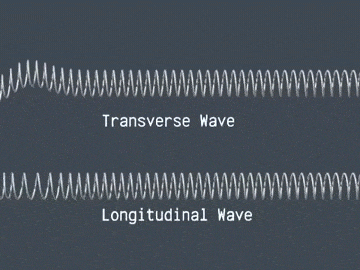## Transverse Waves

Vibrations are at right angles to the motion of the wave. Examples of transverse waves include light, water, and mexican waves!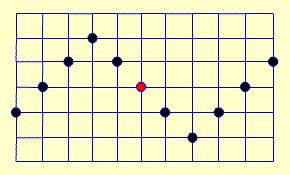Transverse waves have peaks/crests and troughs. The amplitude of the wave is the distance from the central point to a peak or a trough (the maximum displacement of the wave). The wavelength of the wave (given the symbol λ) is the distance from one peak to the next or from one trough to the next.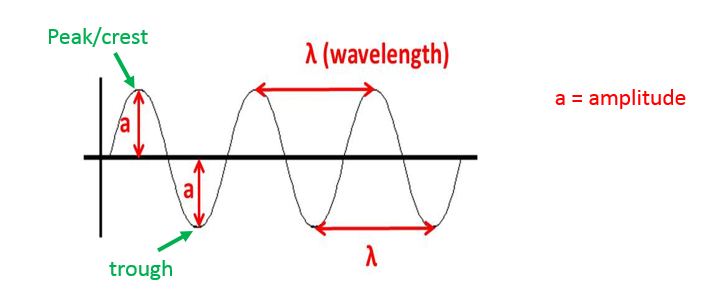## Required Practical – Measuring Waves

Wave features can be measured using a ripple tank. A motor produces waves (ripples) in a shallow tank of water. The tank has a transparent base and a light above it. This allows the waves to be seen as shadows moving on the surface below the tank.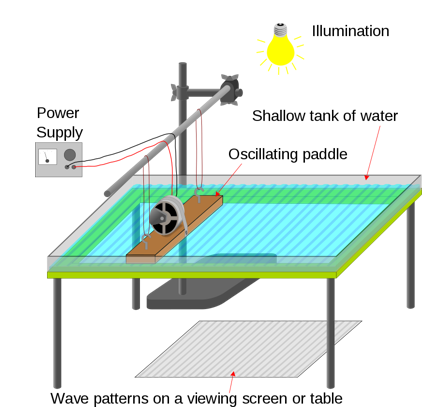The wavelength is the distance between two shadows and the frequency is the number of shadows passing a point each second. This can be difficult to do as the waves/shadows are moving constantly.

Video Ripple tank 1

Video Ripple tank 2

Standing waves can be produced using a piece of string/elastic attached to a vibration generator. As the frequency of the vibrations is adjusted, a standing (stationary) wave can be produced.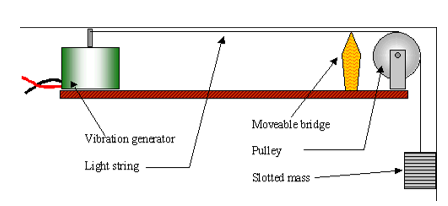The wavelength is the distance across two nodes. The frequency can be measured from the vibration generator itself.

Video Standing Waves 1

Video Standing Waves 2

## Required Practical – Reflection

Reflection from a plane (flat) mirror can be investigated by using a ray box to shine a single ray of light at the mirror. If the mirror and ray box are set up on top of a piece of paper, the position of the mirror, the incident ray (the ray of light travelling from the ray box to the mirror) and the reflected ray (the ray of light that has reflected off the mirror) can be marked on the paper.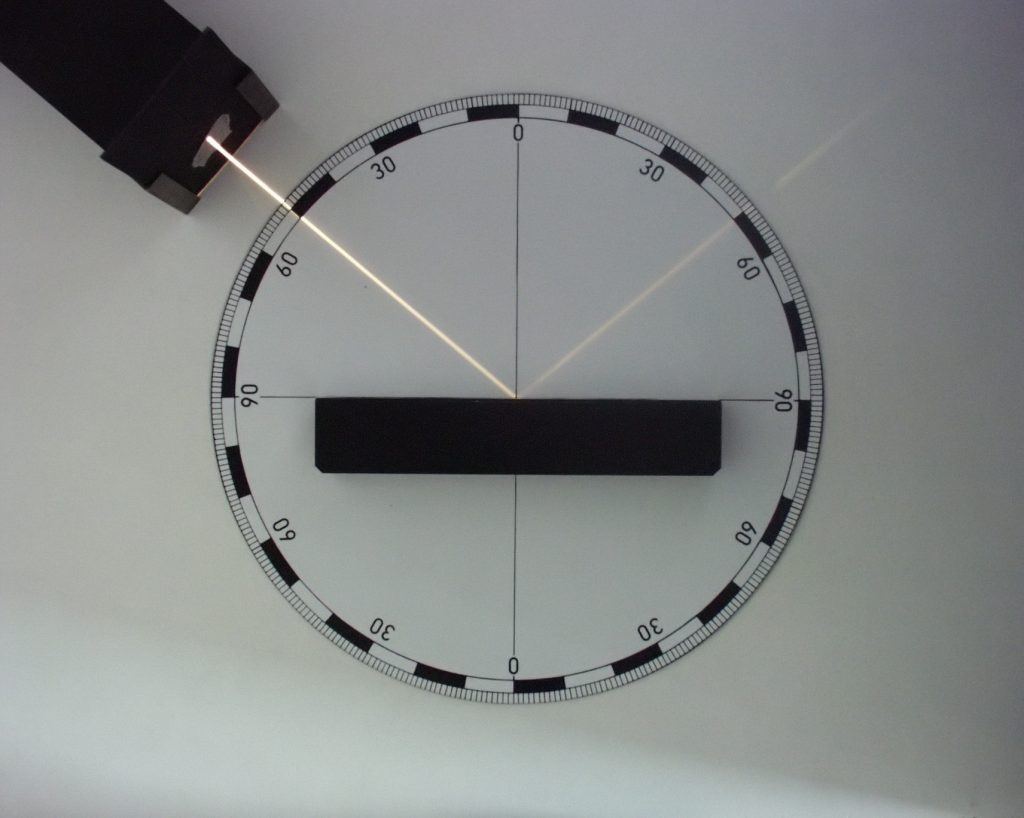With these light rays drawn, a line should be drawn at 90o to the mirror at the position where the ray was reflected. This is called the ‘normal’. The angle of incidence and angle of reflection can then be measured by using a protractor to measure the angle between the ray and the normal.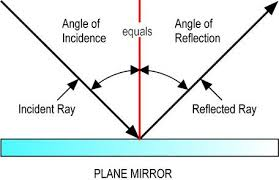The law of reflection states that the angle of incidence is always equal to the angle of reflection.

Video 1

Video 2

## Required Practical – Refraction

The movement of light from one medium (material) to another can be investigated by using a ray box to shine a single ray of through a glass or Perspex block. If the block and ray box are set up on top of a piece of paper, the position of the block and the light rays entering and leaving the block can be marked on the paper.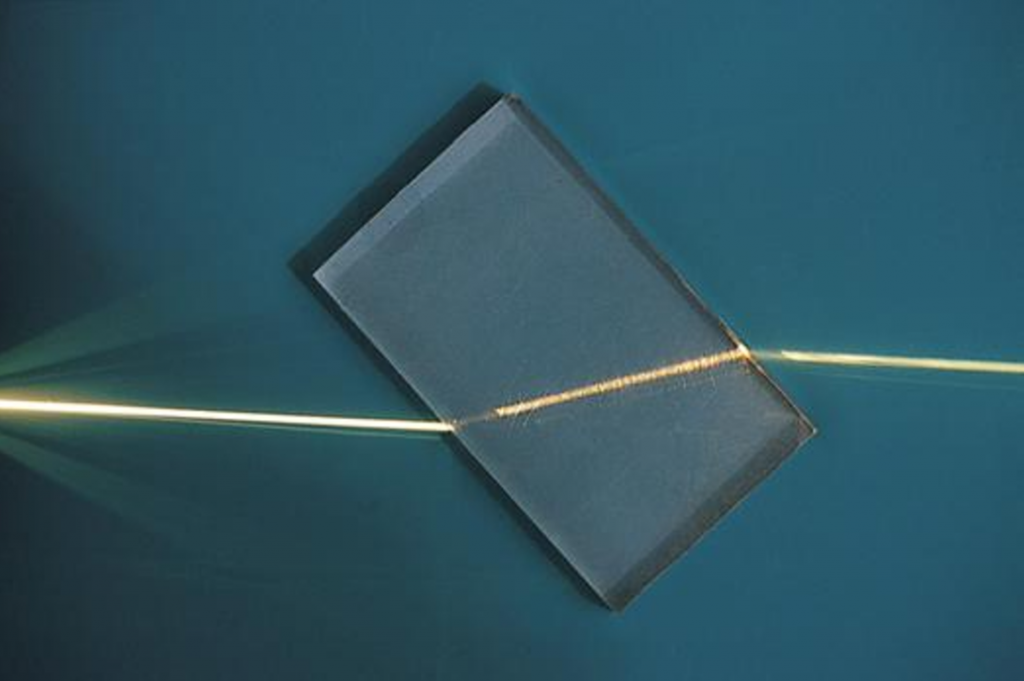If the ray of light enters the block at 90o to the edge of the block, it will pass through the block without changing direction. If the ray enters the block at an angle, it’s path will bend towards the normal as it enters the block and away from the normal when it leaves the block. This is known as refraction and occurs because the light changes speed as it travels from one material to another. (this will be explained in the light lesson)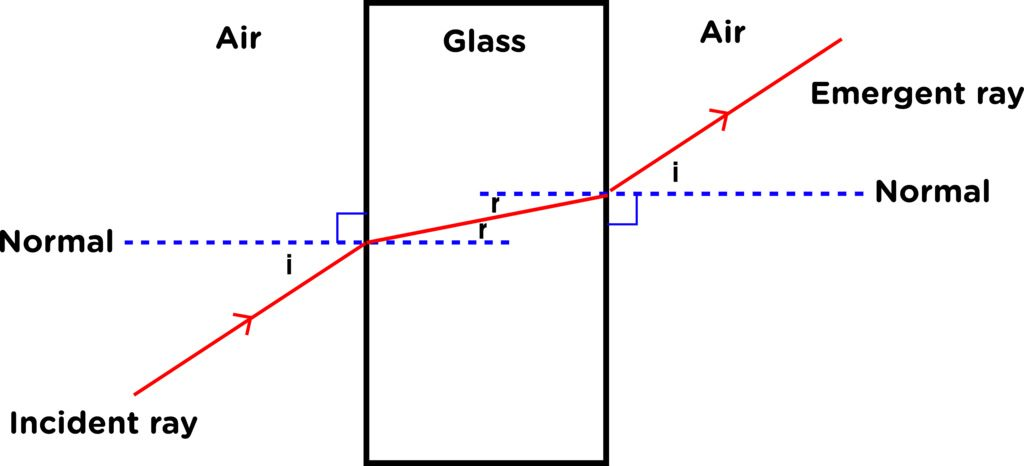Video 1

Video 2

## Longitudinal Waves

Vibrations are in the same direction as the motion of the wave. Sound is an example of a longitudinal wave.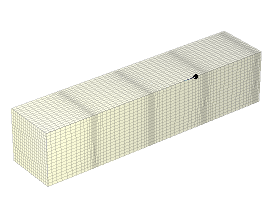Longitudinal waves have compressions (areas where the particles are closer together) and rarefactions (areas where particles are further apart). The wavelength of a longitudinal wave is the distance from one compression to the next.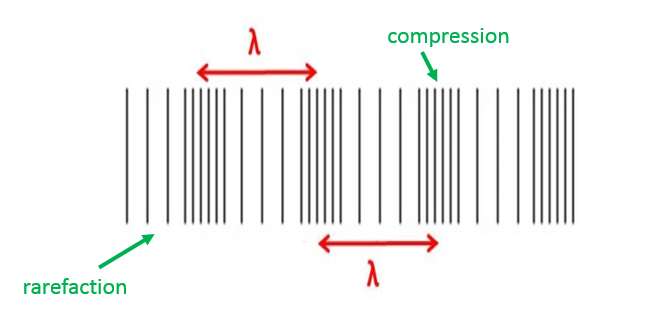Frequency

The frequency of a wave is the number of waves passing a point in 1 second. Frequency is measured in Hertz (Hz).

A wave with a high frequency will have a short wavelength and a low frequency wave will have a long wavelength (provided the speed of the wave is constant).

Period

The period of a wave is the time take for one complete oscillation.

Period = 1 ÷ frequency

Wave Speed

Wave speed is calculated using this equation (you DO need to remember this equation for your exam):

## v = f λ

v = wavespeed (m/s)

__f __= frequency (Hz)

λ = wavelength (m)

## Sound

Sound waves are longitudinal waves that travel through the air from a vibrating object to our ears.

In the ear, the sound wave causes the ear drum to vibrate. These vibrations are converted to an electrical signal which travels to the brain.

The bigger the amplitude of the wave, the louder the sound. The shorter the wavelength of the wave, the higher pitched the sound. High frequency = high pitch.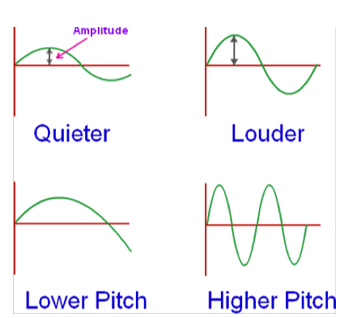The conversion from sound waves to vibrations in a solid (eg the ear drum) only works efficiently over a limited range. Therefore, the range of human hearing is limited

Human hearing range is approximately 20Hz – 20kHz.Different animals have different hearing ranges: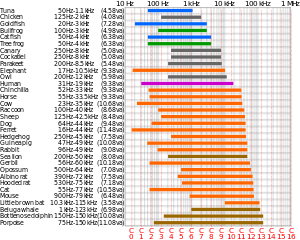## Ultrasound

Sound waves travel at different speeds through different materials. Materials reflect/absorb sound in different ways. These differences can be used to build up a picture of structures which would not normally be visible

Ultrasound is sound above the hearing range of humans (20kHz)

Ultrasound can be used to create an image of a baby in the womb or of other structures in the human body.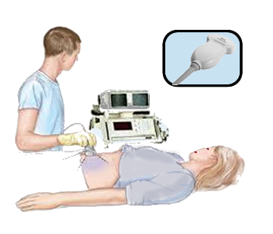Ultrasound travels into the body and some is reflected at the boundary of different tissues

The reflected ultrasound arriving back at the detector at different times can build up a picture of the depth of the different tissues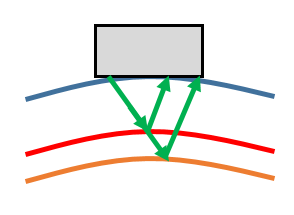1. They can be used to scan organs and other soft tissues (which are not dense enough to absorb X-rays)
2. Ultrasound is non-ionising so there is no risk to the cells in the body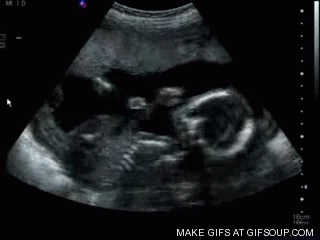## Echo Sounding

Echo sounding uses pulses of high-frequency sound to measure the depth of water or to detect objects in deep water

The time taken for the reflected sound to arrive back at the detector is used to calculate depth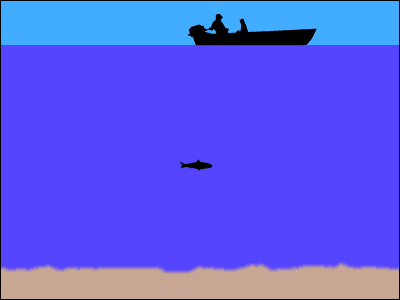## Seismic Waves

The Earth’s structure can be described as 4 parts: the crust, the mantle (molten rock), a liquid outer core and a solid inner core.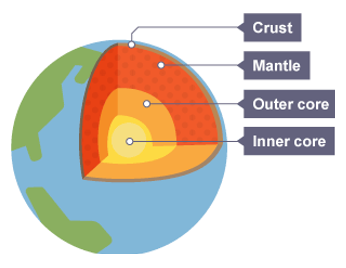This model for the structure of the Earth has been developed using seismic waves – the shock waves produced by earthquakes. There are two main types of seismic waves, P-waves (primary waves) and S-waves (secondary waves)

P-Waves

1. Primary (travel faster, arrive first)
2. Longitudinal Waves
3. Can travel through solid and liquid (core)
4. Cause a one-off ‘impact’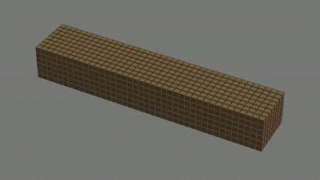S-Waves

1. Secondary (travel slower, arrive second)
2. Transverse Waves
3. Can not travel through liquid (core)
4. Cause shaking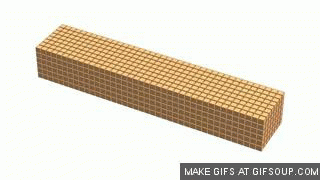Seismic waves travel through the earth after an earthquake. The longer the gap between detecting the P and S waves, the further away the epicentre of the earthquake is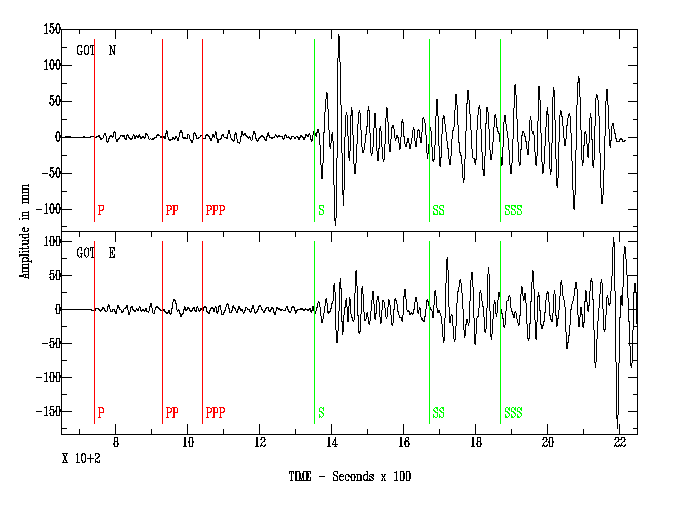Which type of wave has vibrations in the same direction as the motion of the wave?
longitudinal
Which type of wave has vibrations at right angles to the motion of the wave?
transverse
What is the name of the maximum displacement of a wave (distance to peak or trough)?
amplitude
What is the name of the distance from one peak to the next?
wavelength
What units is frequency measured in?